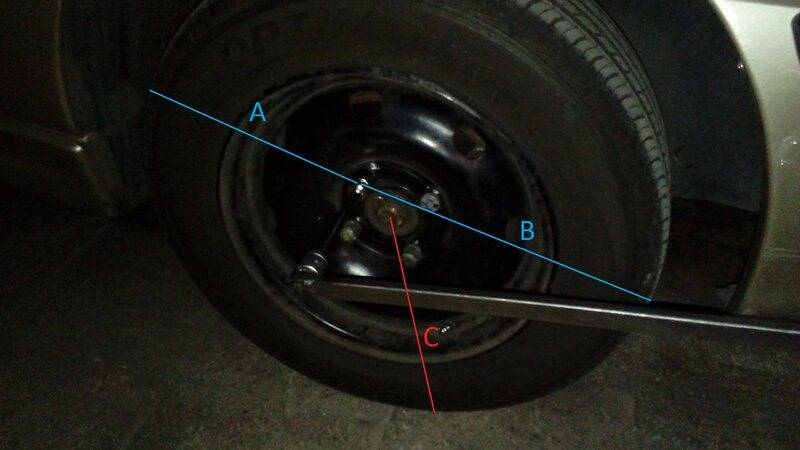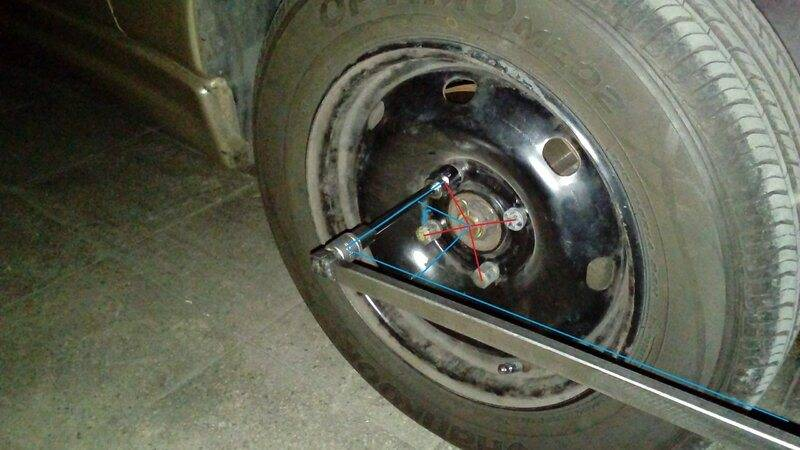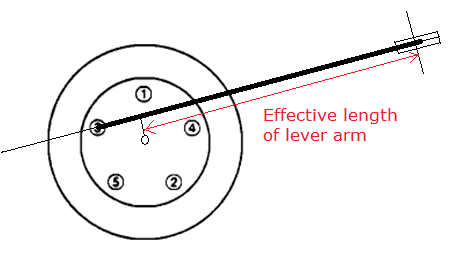# Analyzing applied torque to one of lug nuts instead of wheel center

• karabiner98k

#### karabiner98k

We all know that torque consists of force and distance. If we apply torque to the center of a car wheel, the force that the tire exerts to the ground can be calculated by dividing the torque by tire radius but what about applying torque to one of lug nuts which is off center?In the above picture of my car:

C = Tire radius = 0.289 meter
B = 0.339 meter
A = 0.238 meter

Therefore, B > C > A

If I set my torque wrench to 35 nm and torque the center bolt down, the force would be: 35 / 0.289 = 121 N
What about applying torque to one of lug nuts at the point between B & A?
How do you calculate force if I apply the same 35 nm to it?
35 / 0.339 = 103 N? or 35 / 0.238 = 147 N?
Which one is the answer and why?

Last edited:

Construct a normal to the line of the wrench handle, a normal that passes through the wheel centre. The distance from that normal, along the wrench to your hand, is the effective wrench length when computing torque to the wheel. It may be more or less than the lug nut torque.

Lug nut torque is not my question because I already know the torque which I'm applying to the nut (35 nm). When I apply this amount of torque to the nut, the wheel starts rotating very slowly and the car begins moving forward. So, there is a force that moves it forward and I want to know the magnitude of this force. If the torque was being applied to the center of wheel, the force would be simply obtained by dividing the torque by tire radius but I don't know how to get it in this case since the nut is off-center.

What part of my post do you not understand ?

The picture is too black.

If the nuts are at a radius of r from the centre of the wheel, then the handle of the torque wrench is effectively lengthened or shortened by a maximum of r, depending on the direction of the handle. If the handle passes over the centre of the wheel, the length is reduced by r, so the torque is less. If the handle points away from the wheel centre, then the handle is effectively lengthened by r, so torque is increased.

•karabiner98k
I replaced the black picture with a bright one.Last edited:•hutchphd and karabiner98k
Thanks for your drawing. What about acceleration force that the tire exerts to the ground and causes movement of the car?

What about acceleration force that the tire exerts to the ground and causes movement of the car?
The force applied to the torque wrench handle, Fh, is multiplied by the torque wrench effective length, w, then divided by the wheel radius. That gives the horizontal force applied to the ground; Fg .
Then use F = m a; So, acceleration; a = Fg / m; where m is the mass of the vehicle.

•karabiner98k
Lug nut torque is not my question because I already know the torque which I'm applying to the nut (35 nm). When I apply this amount of torque to the nut, the wheel starts rotating very slowly and the car begins moving forward. So, there is a force that moves it forward and I want to know the magnitude of this force. If the torque was being applied to the center of wheel, the force would be simply obtained by dividing the torque by tire radius but I don't know how to get it in this case since the nut is off-center.
You could do a free body diagram of the wheel and a balance of forces and moments about the contact patch.
Those 35 N-m of torque are applied to the whole wheel, not only to the lug nut.